# How to use count function in excel, include count repeated and unique values

Lionsure 2019-10-25 Original by the website

The Count function is used to count the number of numeric value and date in Excel, it must contain at least one value and at most 255 values. The Count function does not count spaces and text that cannot be converted to numbers. If you want to count them, you need to use the CountA function.

The Count function is often used in combination with the If, Find, and Frequency functions. The Count + If combination can count the number of specified Criteria, the Count + Find combination can count the number of repeated values, and the Count + Frequency combination can count the number of cells that do not include duplicate values (ie excel count unique values).

### I,  Excel Count function syntax

1. Expression: COUNT(Value1, [Value2], ...)

2. Description:

A. Argument value can be a number, an array, a reference to a cell or a range of cells, at least one value, up to 255.

B. If Value is a number, date, and text that can be converted to a numeric value(such as "2"), they are counted as a counted number. If Value is a logical value(True or False), if Value is written directly in the Count function, it is counted; if Value is a value in a cell or array, it is not counted. If you want to count cells with text, logical values, null values ""(such as returned by the formula) and error values, you can use the CountA function.

C. If Value is a space, text that cannot be converted to a numeric value(such as "two"), or an incorrect value, it will not be counted.

### II, How to use count function in excel

(I) The reference range is cells

Suppose you want to count the number of digits in column C. Double-click the cell C8, enter the formula =count(c2:c7), press Enter, return to the counted result 6; the process steps, as shown in Figure 1: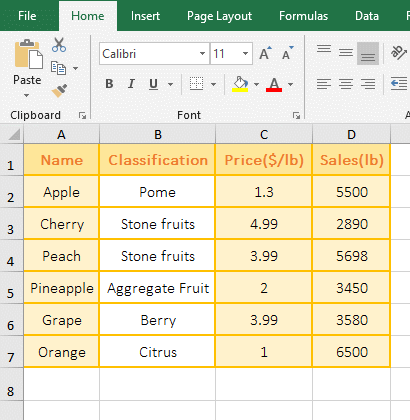Figure 1

(II) Count the value in the array

1. Double-click the cell A1 cell, copy the formula =COUNT(6,{3,8,10,5,2}) to A1, press Enter, return to the counted result 6; the operation steps are as shown in Figure 2:Figure 2

2. The arguments of formula =COUNT(6,{3,8,10,5,2}) are a number and an array, the counted result is 6, indicating independent numbers and arrays are both counted.

(III) Value is a number, date, or text that can be converted to a numeric value

1. Double-click the cell B1, enter the formula =COUNT(A1:A3), press Enter, return to the counted result 2, delete the "16" in A3, the value in B1 is still 2; double-click B2, Copy the formula =COUNT(38,2018/9/8,"16") to B2, press Enter, return to the counted result 3; the operation process steps, as shown in Figure 3: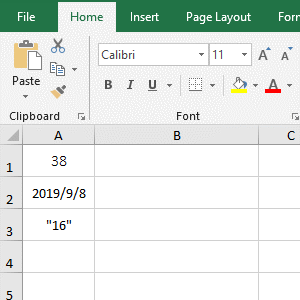Figure 3

2. Three identical values, one formula counted result is 2, another formula counted result is 3, the difference between them is that one value is in the cells, the other value is in the array, and as can be seen from the operation, the "16" in the cell is not counted, and the "16" in the array is counted; this means that "16" in the array is text that can be converted to a numeric value, and "16" in the cell is not, the Text in a cell that can be converted to a numeric value means that the format of cell is textual.

(IV) Value contains logical values True and False

1. Double-click the cell B1, enter the formula = COUNT(A1:A3), press Enter, return to the counted result 1; double-click B2, copy the formula = COUNT(38,TRUE,FALSE) to B2, press Enter, return to counted result 3; operation process steps, as shown in Figure 4: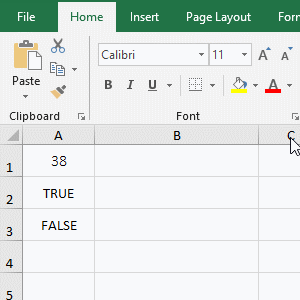Figure 4

2. The two formulas also count the same value, one returns 1 and the other returns 3, indicating that when the logical value is in the cell, the Count function does not count them, but counts them in the array.

(V) Value is empty cells, null values, and error values

1. Double-click the cell B1, copy the formula =COUNT(A1:A4) to B1, press Enter, return to the counted result 0; double-click A1, there is nothing inside, indicating that it is an empty cell; then double-click A2, there is a If formula that returns null value, indicating that the null value is also ignored by the Count function; the operation process steps, as shown in Figure 5: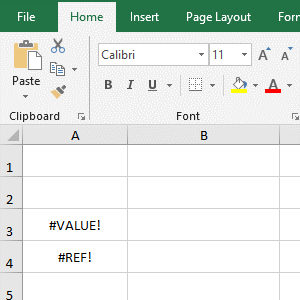Figure 5

2. As can be seen from the operation, the Count function ignores empty cells, null values, and error values.

### III, The Excel Count function extension use case

(I) If + Count function combination to count the number of numeric values that meet the specified Criteria.

1. If you want to count the number of fruits sold with the sales volume greater than 2000. Double-click the D8 cell, copy the formula =COUNT(IF(D2:D7>2000, D2:D7,"")) to D8, press Enter, return to the counted result 5; the operation steps, as shown in Figure 6: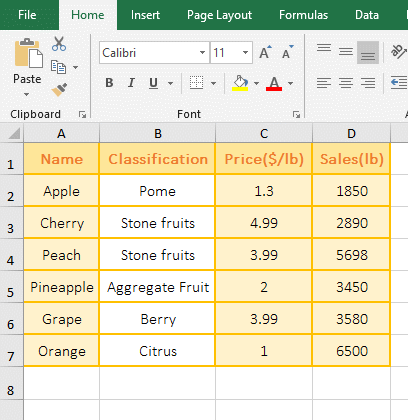Figure 6

2. The formula =COUNT(IF(D2:D7>2000, D2:D7,"")) description:

A. The formula is an array formula, so press Ctrl + Shift + Enter.

B. D2:D7>2000 is the Criteria of If, D2:D7 returns the values in D2 to D7 as an array, and then each element is taken out from the array and compared with 2000, if it is greater than 2000, it returns True, otherwise it returns False; for example: Take out 1850 for the first time, return FALSE, because it is not greater than 2000; take out 2890 for the second time, because it is greater than 2000, return TRUE, and so on; and finally returning {FALSE;TRUE;TRUE;TRUE;FALSE;TRUE}.

C. Then IF(D2:D7>2000,D2:D7,"") becomes IF({FALSE;TRUE;TRUE;TRUE;FALSE;TRUE},D2:D7,""), then, take the first element FALSE from the array, because it is false, returns ""; take the second element TRUE from the array for the second time, since it is true, it returns the element D3 that is the corresponding elements of D2:D7 and the array; the others and so on; Returns {"";2890;5698;3450;3580;6500}.

D. The formula becomes =COUNT({"";2890;5698;3450;3580;6500}), and finally Count is used to count the number of values in the array, so the result is 5.

(II) Count + Find function combination to count the number of repeated values

1. If you want to count the number of "Stone fruits" in the "Classification" in the fruit table. Double-click the cell B8, copy the formula =COUNT(FIND("Stone fruits", B2:B7)) to B8, press Ctrl + Shift + Enter to return to the counted result 2. The process steps are as shown in Figure 7: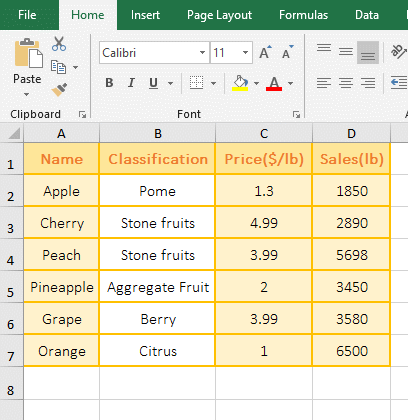Figure 7

2. The formula =COUNT(FIND("Stone fruits", B2:B7)) description:

A. FIND("Stone fruits", B2:B7) is used to find all "Stone fruits" in B2:B7, if it is "Stone fruits", returns 1, otherwise returns error #VALUE!, and finally returns the array {#VALUE !;1;1;#VALUE!;#VALUE!;#VALUE!}.

B. The formula becomes =COUNT({#VALUE !;1;1;#VALUE!;#VALUE!;#VALUE!}), and finally the array is counted. Since the error value is not counted, only the value is counted, so the counted result is 2.

(III) Excel count unique: Count + Frequency function combination to count the number of values that are not repeat

1. If you want to count the price that do not include the number of duplicates. Double-click the cell C8, copy the formula =COUNT(0/(FREQUENCY(C2:C7,C2:C7))) to C8, press Enter, return to the counted result 5, and operate the process steps, as shown in Figure 8: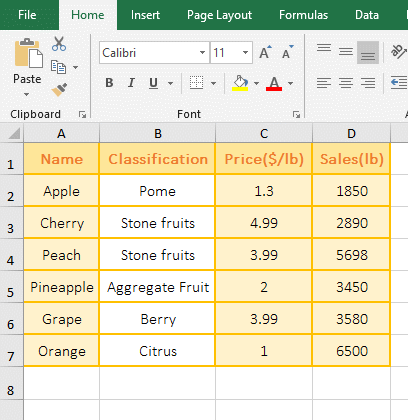Figure 8

2. The formula =COUNT(0/(FREQUENCY(C2:C7,C2:C7))) description:

A. FREQUENCY(C2:C7,C2:C7) is used to count the frequency of occurrence of each value in C2:C7; when executed, C2(ie 1.3) in the second argument is taken out, then Count the number of value(the result is 2) less than or equal to 1.3 and greater than 1 in C2:C7, because C2:C7 is directly used as the argument Bins_Array of the Frequency function (that is, the interval argument is divided into the value to be counted), and the value smaller than 1.3 is 1, therefore, 1 and 1.3 are an interval;

C3(ie 4.99) in the second argument is taken out for the second time, and the number of value(result is 1) less than or equal to 4.99 and greater than 3.99 is also counted in C2:C7; take out 3.99 for the third time, and the number of values(result is 2) less than or equal to 3.99 and greater than 2 are also counted in C2:C7; take out 3.99 for the fifth time, since 3.99 has been counted and no longer counts, so the result is 0; the others and so on, and return finally the array {1;1;2;1;0;1;0}.

B. Then 0/(FREQUENCY(C2:C7,C2:C7) becomes 0/{1;1;2;1;0;1;0}, then divide 0 by each number in the array, the result is {0;0;0;0;#DIV/0!;0;#DIV/0!}; Dividing 0 by each value in the array is mainly to filter out the 0 in the array, that is, the repeated and no values are filtered out.

C. The formula becomes =COUNT({0;0;0;0;#DIV/0!;0;#DIV/0!}), and finally the values in the array are counted, there are 5 0 in total in the array, the two divisor is 0 error #DIV/0!, so the result is 5.